．原載於科學月刊十六卷第一期 ．作者當時任教於台大數學系 •註釋 代數學基本定理 曹亮吉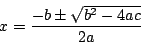(u+v)3-3uv(u+v)-(u3+v3)=0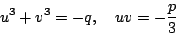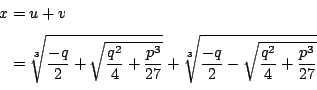(1)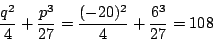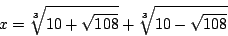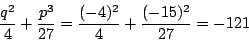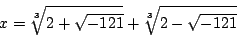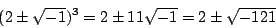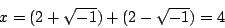x-4 這個因式從 x3-15x-4 除去後，得商 x2+4x+1。所以 x3=15x+4 還有 x2+4x+1=0 的兩個解。然而它們在 Cardano 的解法中怎麼沒有出現？ 毛病在於把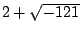開三次方時，我們只取其中的一個根。如果以 w 表 1 的三次方根，則的其他兩個三次方根為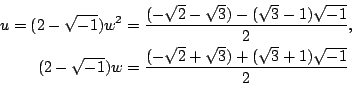(2)

f(z)=p(x,y)+q(x,y)i

p(x,y)q(x,y) 都是實數，都是 x,y 的函數。求 f(x)=0 的根就等於求 p(x,y)=0, q(x,y)=0 的共解。p(x,y)=0q(x,y)=0xy 平面上各代表一條曲線，所以 Gauss 的主要論點就是在說明這兩條曲線一定會有交點的；這個交點所對應的 z 值，自然就是 f(z)=0 的根了。

Gauss 的第二個證明用的是兩個多項式的結式 (resultant)。若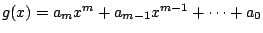為兩個多項式，則行列式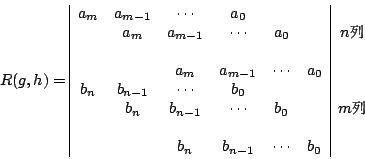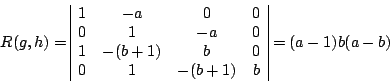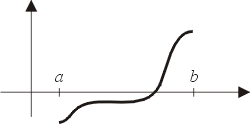圖一：f(a)f(b)< 0，所以f(x)=0有解。

n 為偶數，且只含 2 的一次因數時，Gauss 考慮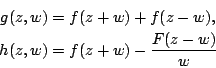Gauss 的第三個證明，用的是複變函數的積分理論，相當深入，在此略過不表。

Gauss 對代數學基本定理所給的證明是劃時代的。從希臘以來，數學的實體必先找到，才能談它的性質；也就是說證明的過程必須從已知的量出發，一步一步作出中間過程的補助量，以達到最後所要的量。這是所謂建構型的證明。 Gauss 的方法卻不按步就班，他只用學理肯定所要量的存在性，而所要的量是否按步就班可得，卻不是證明所關心的，這是一種存在型的證明。

Cardano
Euler
d'Alembert
Lagrange
Wallis
Gauss

 附錄：另一種證法（高中生也能懂的）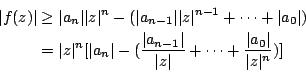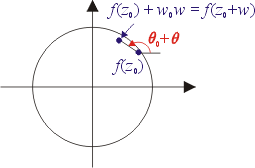圖二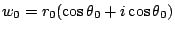,，則（若有指正、疑問……，可以在此 留言 或 寫信 給我們。）EpisteMath (c) 2000 中央研究院數學所、台大數學系 各網頁文章內容之著作權為原著作人所有
 編輯：洪瑛 ／ 繪圖：簡立欣 最後修改日期：3/19/2002